# Python基础(函数)函数的定义格式:

``````def 函数名():
函数代码``````

### 使用当前文件的函数

``````def hello():
print('hello')``````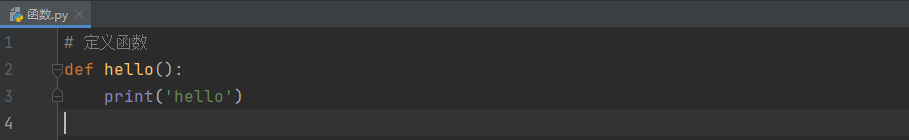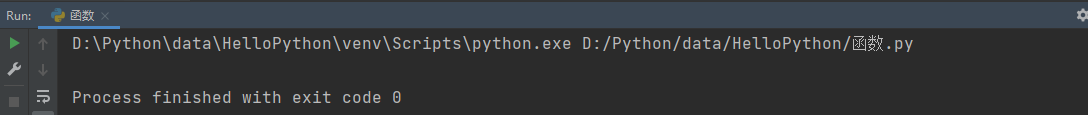``````# 定义函数
def hello():
print('hello')

# 调用函数
hello()``````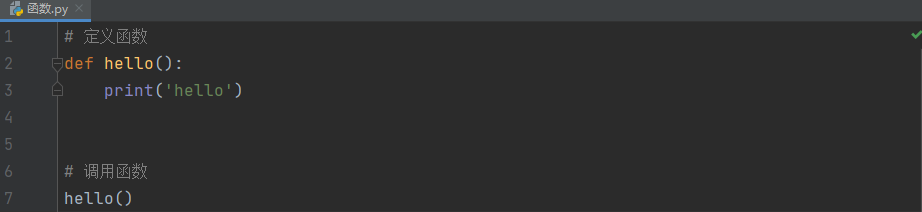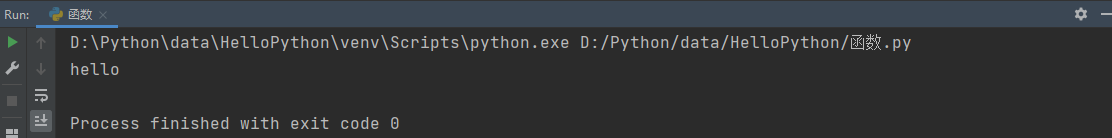需要注意的是, 在有些语言中, 函数的调用可以发生在函数的定义之前, 而Python中, 函数的调用只能在函数的蒂尼之后, 如果在函数定义前就调用函数, Python会找不到函数, 从而报错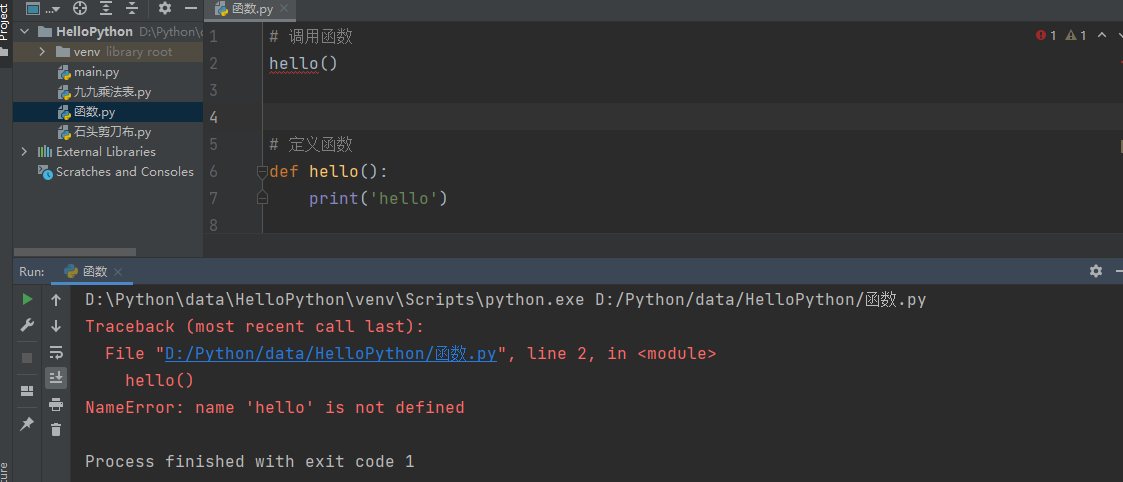### 使用其他文件(模块)的函数

``````# 在控制台连续输出五行*, 每一行星星的数量以此递增
def print_99():
# 定义行数
row = 1
# 循环9次,打印9行
while row <= 9:
# 定义列数
col = 1
while col <= row:
# 列每循环一次,打印 row * col = (row * col), 并取消换行
print('%d * %d = %d  ' % (row, col, row * col), end='')
# 列数递增
col += 1
# 行每循环一次,打印一个换行
print('')
# 行数递增
row += 1``````

``````# 导入函数所在的类(模块)
import 九九乘法表

# 调用函数:模块名.函数名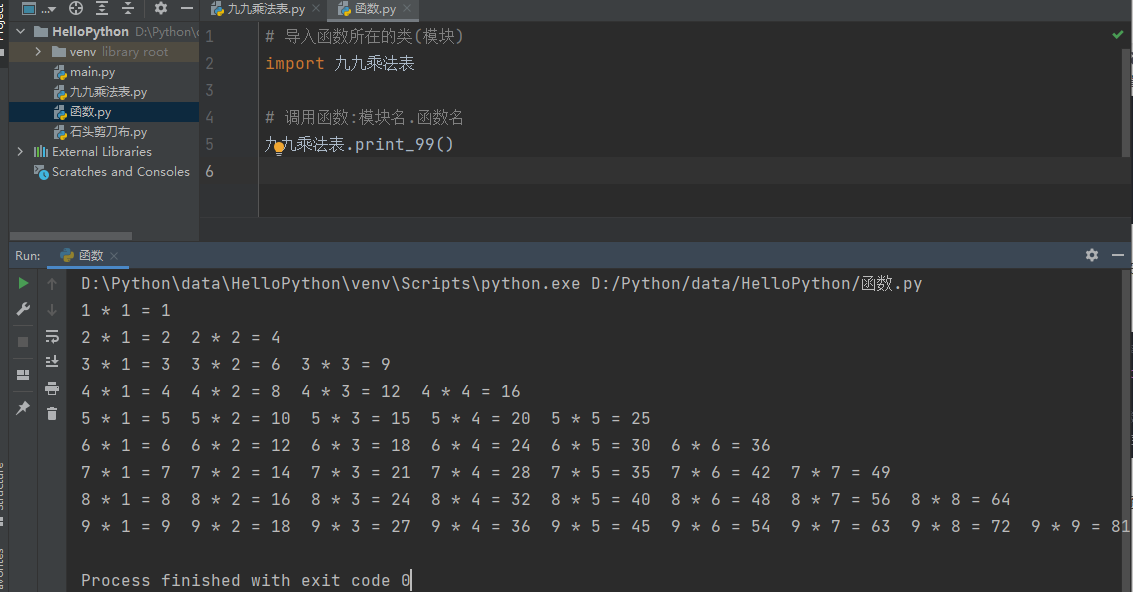### 求两数之和

``````# 定义求和函数
print('%d + %d = %d' % (num1, num2, num1 + num2))

# 调用求和函数

调用函数时, 在参数中传入需要操作的数字即可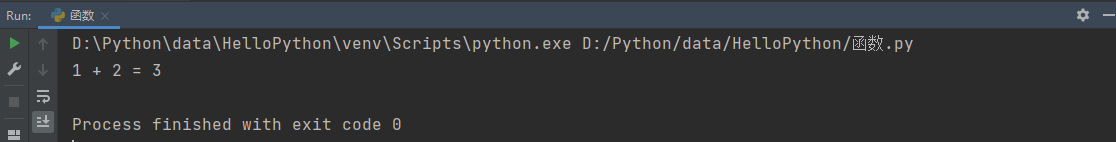-- 展开阅读全文 --

BUUCTF Web [极客大挑战 2019]Knife
« 上一篇 06-24